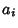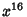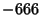## Integer Relation

A set of Real Numbers, ...,is said to possess an integer relation if there exist integerssuch thatwith not all. An interesting example of such a relation is the 17-Vector (1,,, ...,) with, which has an integer relation (1, 0, 0, 0,, 0, 0, 0,, 0, 0, 0,, 0, 0, 0, 1), i.e.,This is a special case of finding the polynomial of degreesatisfied by.

Algorithms for finding integer relations include the Ferguson-Forcade Algorithm, HJLS Algorithm, LLL Algorithm, PSLQ Algorithm, PSOS Algorithm, and the algorithm of Lagarias and Odlyzko (1985). Perhaps the simplest (and unfortunately most inefficient) such algorithm is the Greedy Algorithm. Plouffe's Inverse Symbolic Calculator'' site includes a huge database of 54 million Real Numbers which are algebraically related to fundamental mathematical constants.

See also Constant Problem, Ferguson-Forcade Algorithm, Greedy Algorithm, Hermite-Lindemann Theorem, HJLS Algorithm, Lattice Reduction, LLL Algorithm, PSLQ Algorithm, PSOS Algorithm, Real Number, Lindemann-Weierstraß Theorem

References

Bailey, D. and Plouffe, S. Recognizing Numerical Constants.'' http://www.cecm.sfu.ca/organics/papers/bailey/.

Lagarias, J. C. and Odlyzko, A. M. Solving Low-Density Subset Sum Problems.'' J. ACM 32, 229-246, 1985.

Plouffe, S. Inverse Symbolic Calculator.'' http://www.cecm.sfu.ca/projects/ISC/.